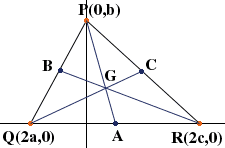Name: Jessica Who is asking: Student Level: Secondary Question: Use a scalene triancle with one point (0,b) on the y axis, another point (2c, 0) on the x-axis and the last point, (2a,0) on the x-axis. Prove that the medians of triangle PQR meet in a central point G. On this problem I have gotten to the point where I have found the Slope of line PA and line RB in y=mx+b form and then I substituted one y in for the other. Now what do I do?? Hi Jessica, After you substitute as you said above you need to solve for x. This gives the x-coordinate of the point G where the lines PA and RB meet. Substitute this value of x into the equation for the line PA and this will give you the y-coordinate of G.What remains is to show that G lies on the line QC. To do this write the equation of the line QC and substitute the coordinates of G into the equation to verify that G is on the line QC. I hope this helps, Penny Go to Math Central